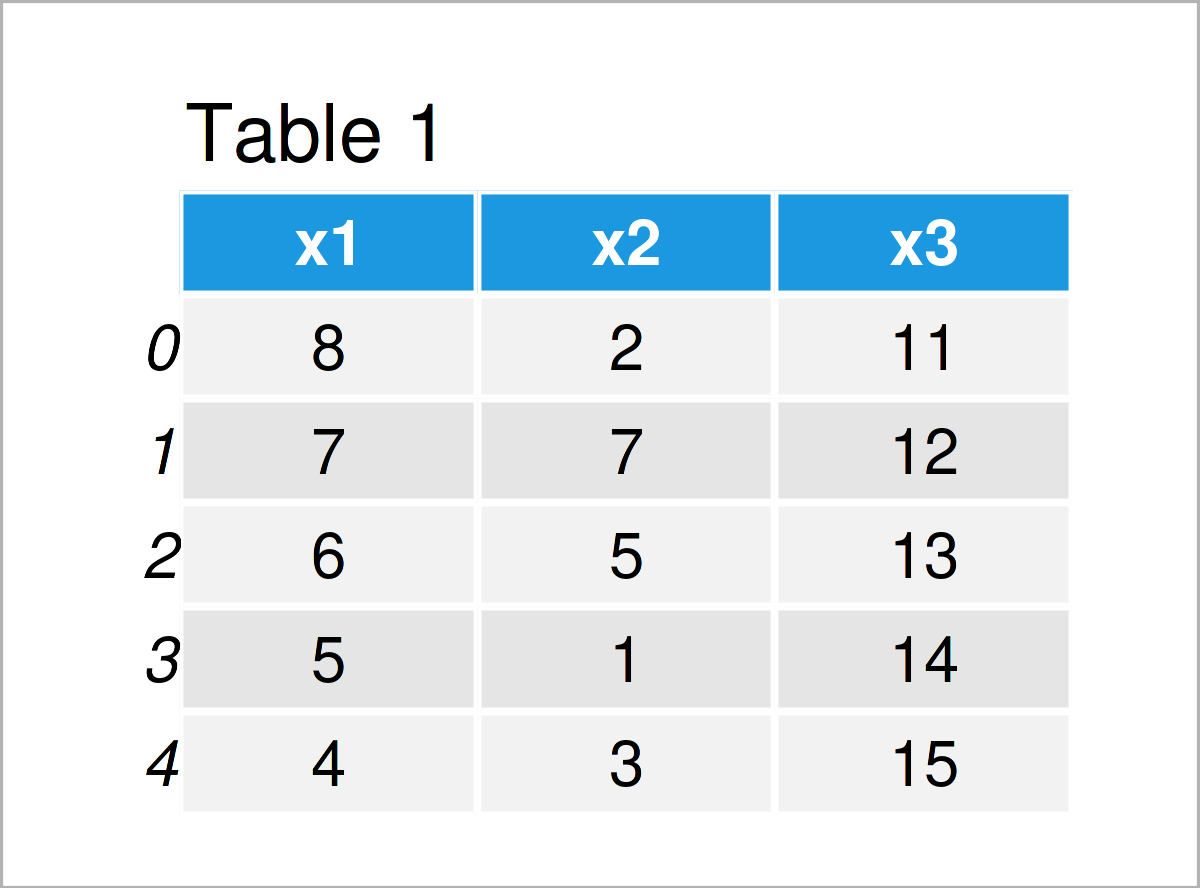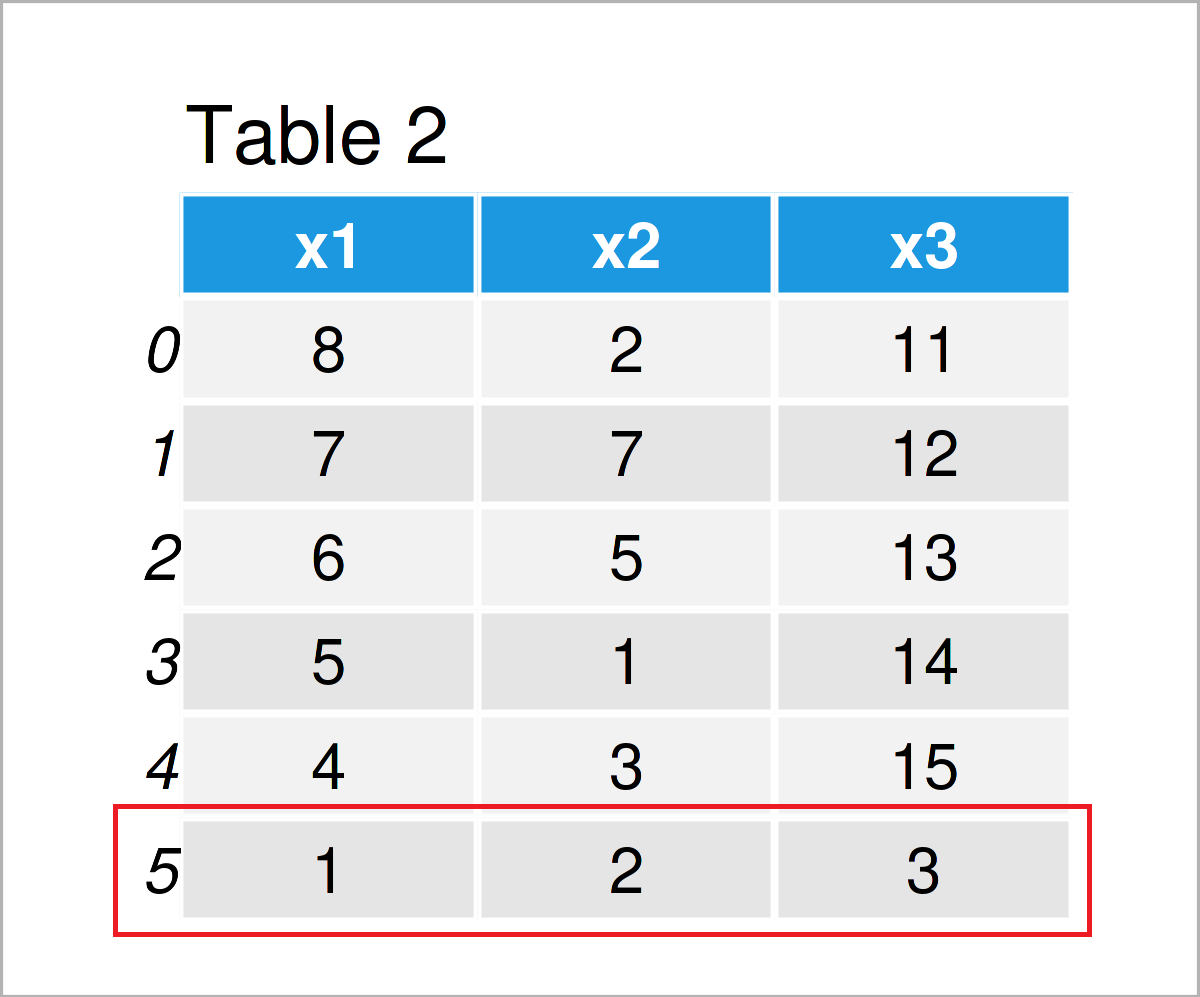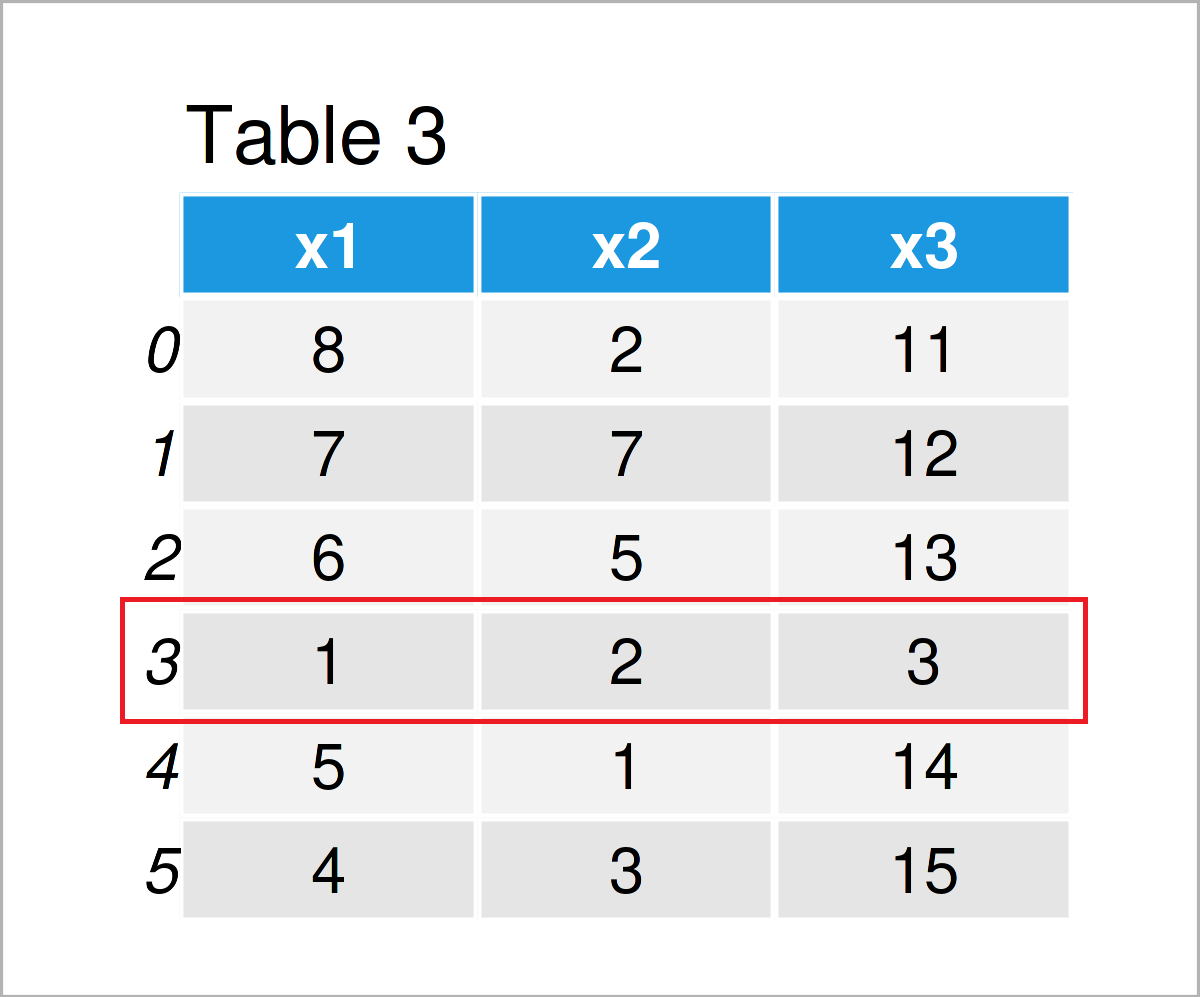# Add Row to pandas DataFrame in Python (2 Examples)

In this tutorial, I’ll show how to append a new row to a pandas DataFrame in Python programming.

The content of the post looks as follows:

Let’s take a look at some Python codes in action:

## Example Data & Libraries

First, we need to load the pandas library:

`import pandas as pd # Import pandas library to Python`

The data below will be used as basement for this Python programming language tutorial:

```data = pd.DataFrame({'x1':range(8, 3, - 1), # Create example DataFrame 'x2':[2, 7, 5, 1, 3], 'x3':range(11, 16)}) print(data) # Print example DataFrame```Table 1 shows that our example data consists of five rows and the three variables “x1”, “x2”, and “x3”.

Next, we have to create a list that we can insert as a new row to our DataFrame later on:

```new_row = [1, 2, 3] # Create new row print(new_row) # Print new row # [1, 2, 3]```

As you can see, our new row that we will combine with our DataFrame contains the values 1, 2, and 3.

## Example 1: Append New Row at Bottom of pandas DataFrame

In this example, I’ll explain how to append a list as a new row to the bottom of a pandas DataFrame.

For this, we can use the loc attribute as shown below:

```data_new1 = data.copy() # Create copy of DataFrame data_new1.loc = new_row # Append new row print(data_new1) # Print updated DataFrame```As shown in Table 2, the previous Python programming syntax has created a new pandas DataFrame with an additional row in the last line of our data.

## Example 2: Insert New Row in the Middle of pandas DataFrame

In this section, I’ll show how to insert a new row within a pandas DataFrame.

Again, we can use the loc attribute for this task. However, this time, we have to specify a value in between the indices of our input DataFrame.

As you can see below, we are using the index position 2.5 to add a new row in the middle of our data.

Furthermore, we use the sort_index and reset_index functions to reset the indices of our new DataFrame (note that this step is optional):

```data_new2 = data.copy() # Create copy of DataFrame data_new2.loc[2.5] = new_row # Insert new row data_new2 = data_new2.sort_index().reset_index(drop = True) # Reset index print(data_new2) # Print updated DataFrame```By running the previous Python programming code, we have created Table 3, i.e. another pandas DataFrame with a new row in the middle of the data.

## Video & Further Resources

Do you need further info on the Python programming syntax of this article? Then I recommend having a look at the following video on the YouTube channel of Ryan Noonan. He’s explaining how to concatenate rows to a pandas DataFrame in another example in the video:

Please accept YouTube cookies to play this video. By accepting you will be accessing content from YouTube, a service provided by an external third party.If you accept this notice, your choice will be saved and the page will refresh.

Furthermore, you could read the related articles on this website:

Summary: You have learned in this article how to concatenate and stack a new row to a pandas DataFrame to create a union between a DataFrame and a list (i.e. rbind) in the Python programming language. Let me know in the comments section below, in case you have any further questions.

Subscribe to the Statistics Globe Newsletter